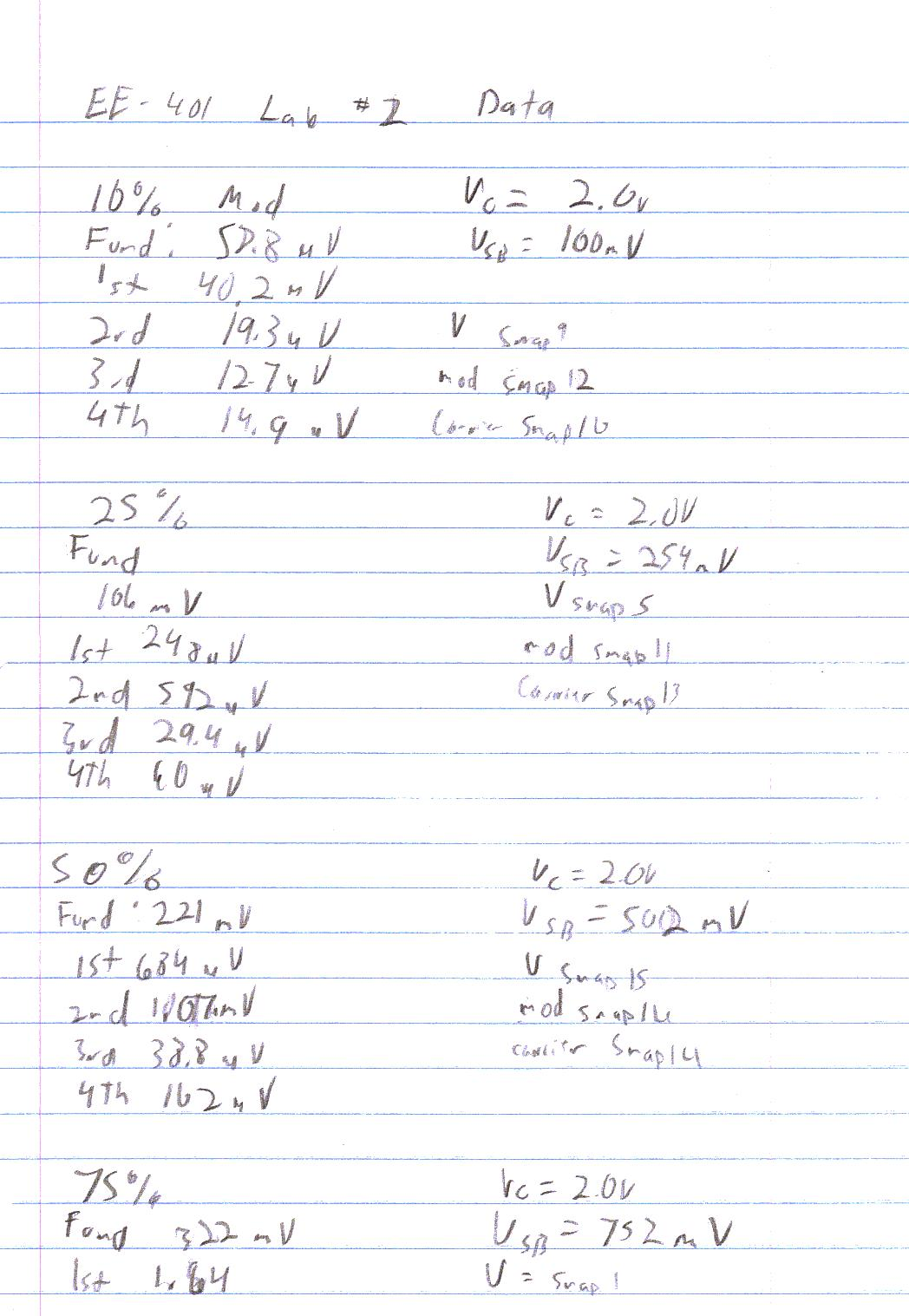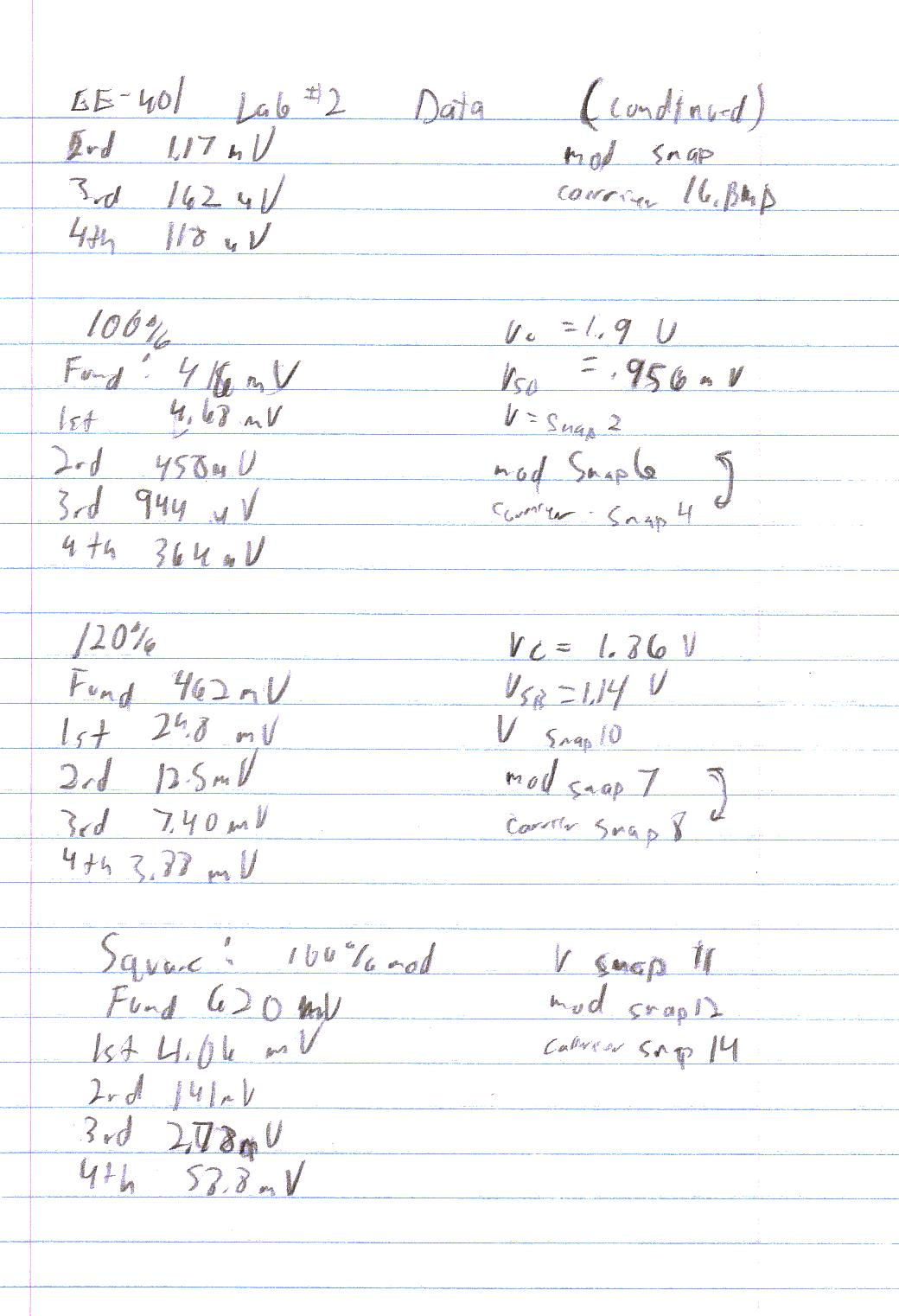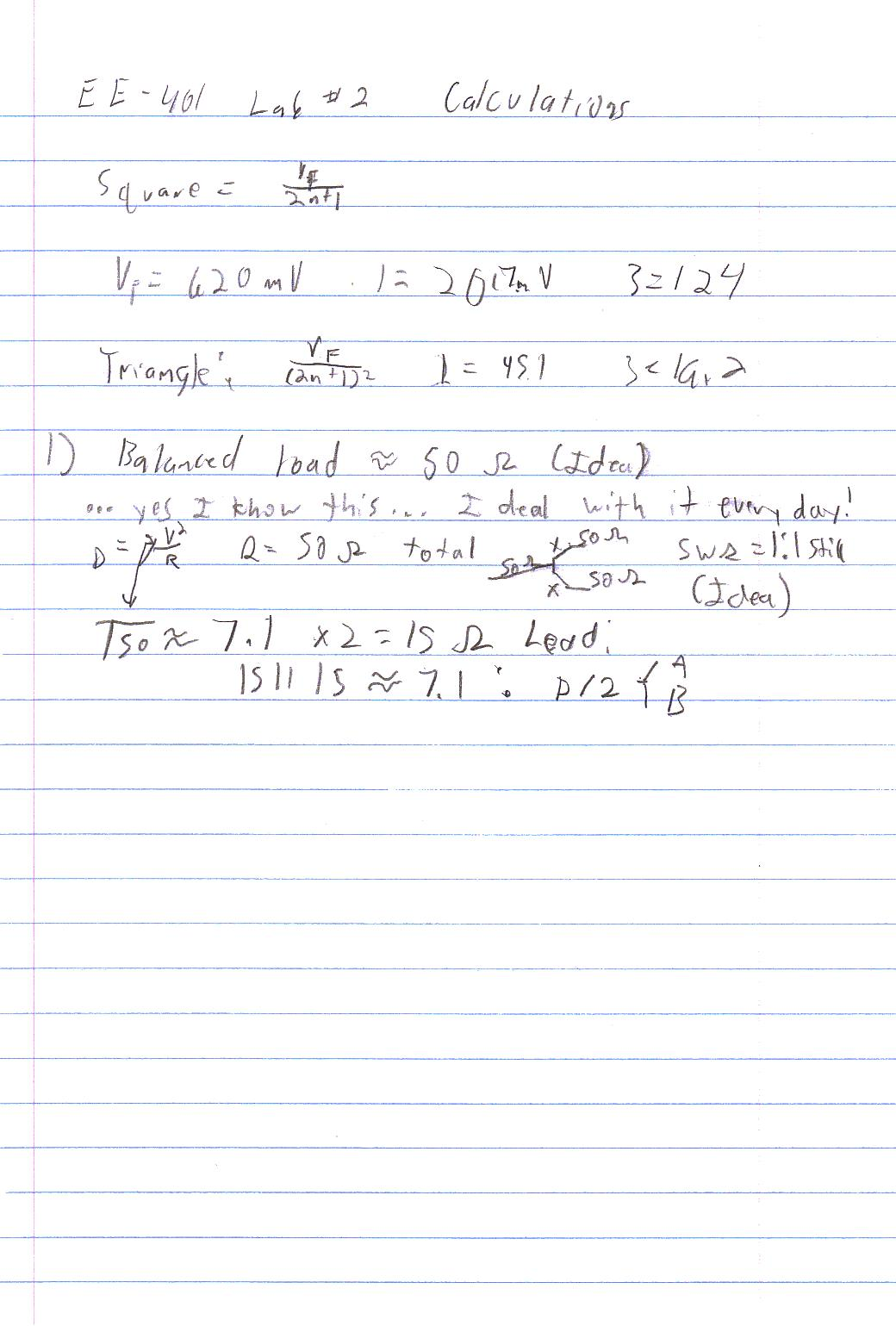EE - 401 / E01 Fall 2002

Introductory Communications Theory

Professor: Conner

Submitted By:

Andrew Buettner

Lab #2: Amplitude Modulation

Thursday, March 06, 2003

1) Cover Page 1

3) Objective 4

4) Components Used 4

5) Procedures 4

6) Lab Data / Results 5

1) Diagram 1 5

2) Diagram 2 5

3) Diagram 3 6

4) Diagram 4 6

5) Diagram 5 7

6) Diagram 6 7

7) Diagram 7 8

8) Diagram 8 8

9) Diagram 9 9

10) Diagram 10 9

11) Diagram 11 10

12) Diagram 12 10

13) Diagram 13 11

14) Diagram 14 11

15) Diagram 15 12

16) Diagram 16 12

17) Diagram 17 13

18) Diagram 18 13

19) Diagram 19 14

20) Diagram 20 14

21) Table 1 15

22) Diagram 21 15

23) Diagram 22 16

24) Table 2 16

25) Diagram 23 17

26) Table 3 17

27) Diagram 24 18

28) Diagram 25 18

7) Answers to Lab Questions 19

8) Conclusions 19

8) Attachments 20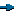Objective

The objective of this lab is to gain in understanding of the significance of modulation bandwidth and index in AM or DSB-FC modulation by generating several different modulation patterns with several different modulation indexes and then calculated the amount of noise that is introduced into each signal.Components Used

1) AF Spectrum Analyzer #1663

2) RF Spectrum Analyzer #1939

3) Function Generator #1510

4) Modulating Function Generator #1511

5) Various components from the EE-301/401 Lab Kit

6) Oscilloscope #1663

7) Trainer #50715653Procedures

1) Assemble the following circuit: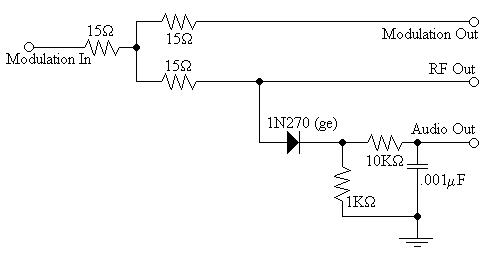2) Apply a DSB-FC signal to the input.

3) Set the AM signal to have a FC of 1MHz and FM of 10KHz.

4) Set the output to 10Vp-p.

5) Connect the oscilloscope channel one to the RF output.

6) Connect the oscilloscope channel two to the demodulated output.

7) Modulate the signal with a modulation index of 10%

8) Show the output waveform and spectrum.

9) Calculate the total harmonic distortion that appears at the output.

10) Repeat steps 7 - 9 using a modulation index of 25%

11) Repeat steps 7 - 9 using a modulation index of 50%

12) Repeat steps 7 - 9 using a modulation index of 75%

13) Repeat steps 7 - 9 using a modulation index of 100%

14) Repeat steps 7 - 9 using a modulation index of 120%

15) Change the modulation signal to a 50% duty cycle , 5KHz square wave with a modulation index of 100%.

16) Sketch the input and output waveforms.

17) Calculate the expected size of the spectral lines.

18) Compare the size of the spectral lines with the observed ones.

19) Repeat steps 15 - 18 using a triangle wave.Lab Data / Results

1) Diagram 1: Schematic (Enlarged View)2) Diagram 2: Voltage / Time view of 10% Modulation:

3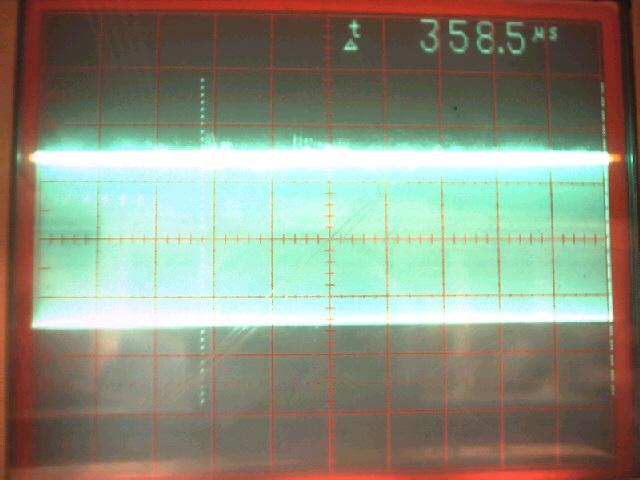) Diagram 3: Voltage / Frequency View of 10% Modulation: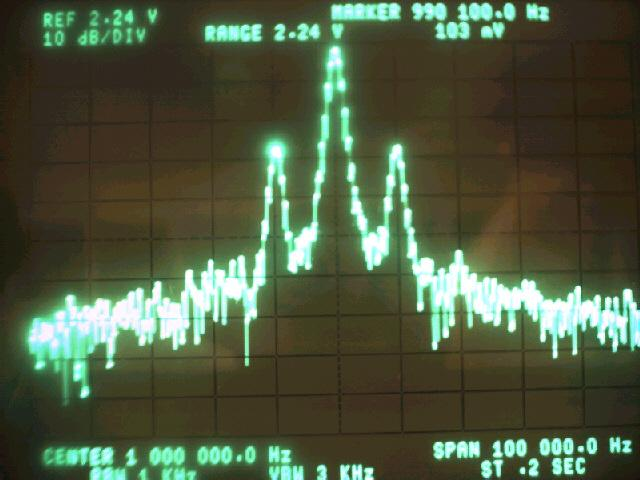4) Diagram 4: Voltage / Frequency View of Demodulated Output:5) Diagram 5: Voltage / Time View of 25% Modulation: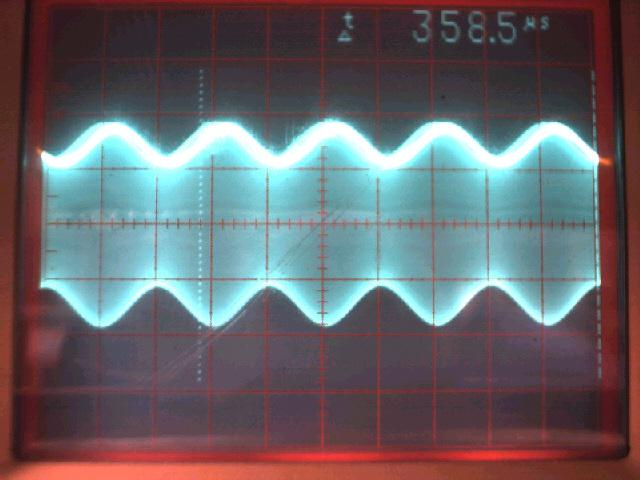6) Diagram 6: Voltage / Frequency View of 25% Modulation:7) Diagram 7: Voltage / Frequency View of Demodulated Output: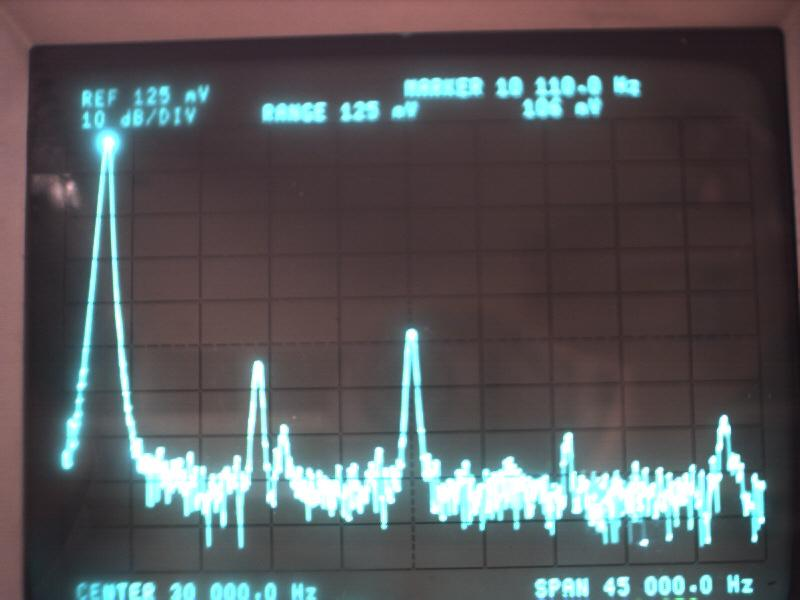8) Diagram 8: Voltage / Time View of 50% Modulation: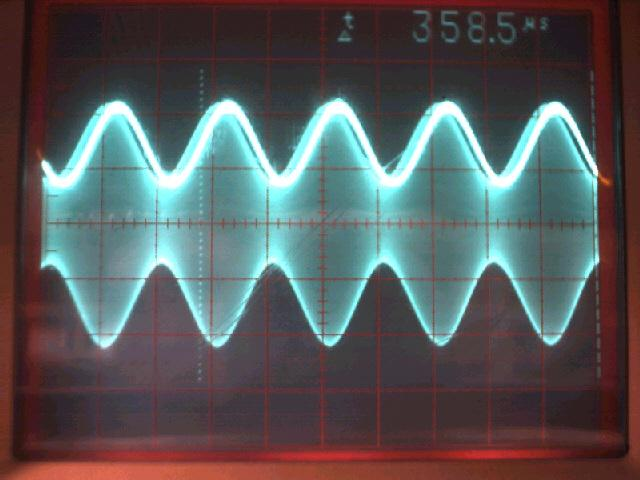9) Diagram 9: Voltage / Frequency View of 50% Modulation:10) Diagram 10: Voltage / Frequency View of Demodulated Output: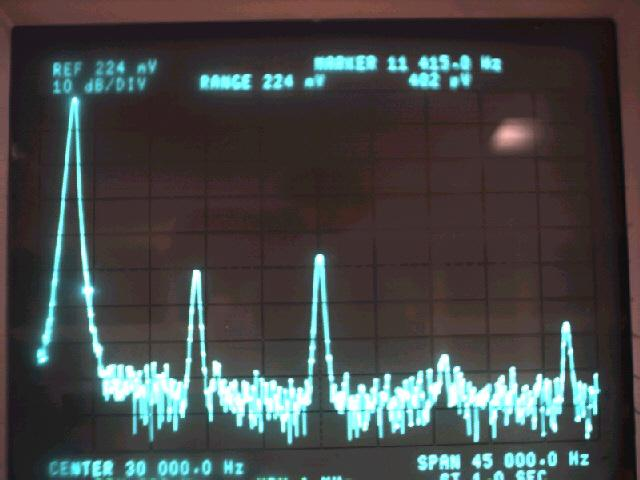11) Diagram 11: Voltage / Time View of 75% Modulation:12) Diagram 12: Voltage / Frequency of 75% Modulation:13) Diagram 13: Voltage / Frequency View of Demodulated Output: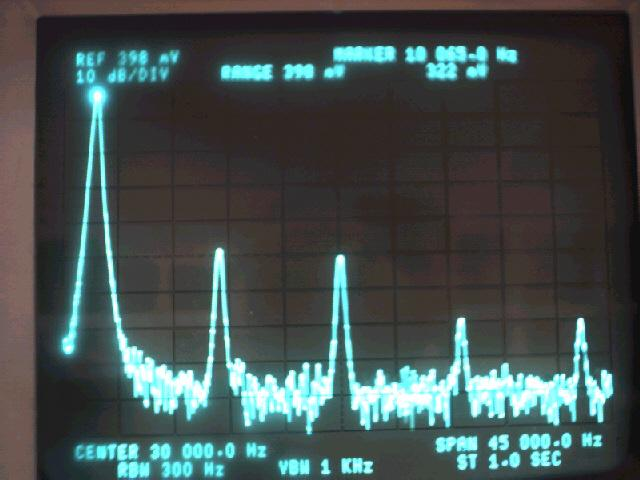14) Diagram 14: Voltage / Time View of 100% Modulation: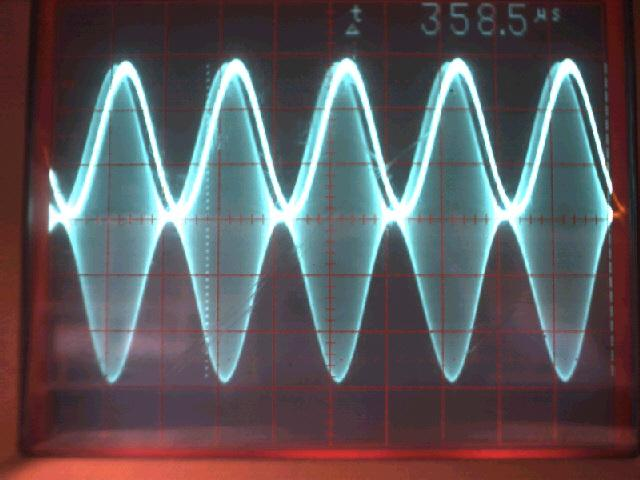15) Diagram 15: Voltage / Frequency View of 100% Modulation: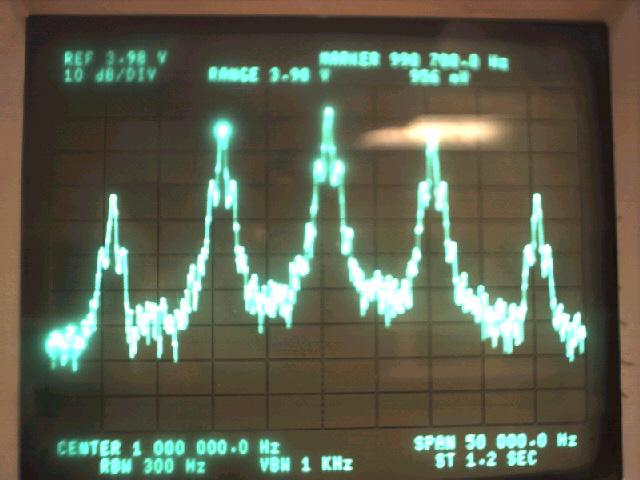16) Diagram 16: Voltage / Frequency View of Demodulated Output: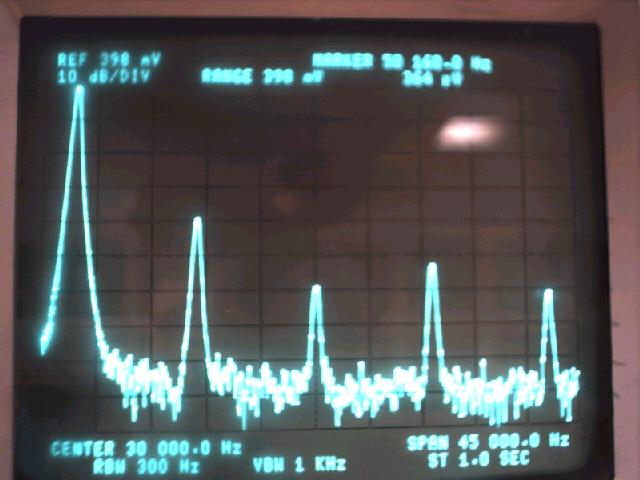17) Diagram 17: Voltage / Time View of 120% Modulation: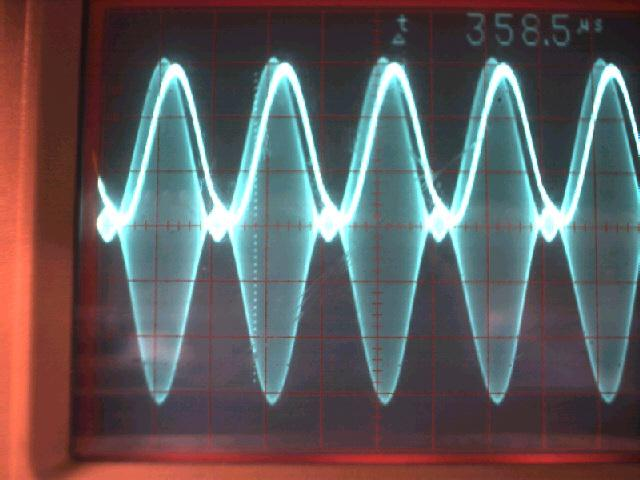18) Diagram 18: Voltage / Frequency View of 120% Modulation:19) Diagram 19: Voltage / Frequency View of Demodulated Output: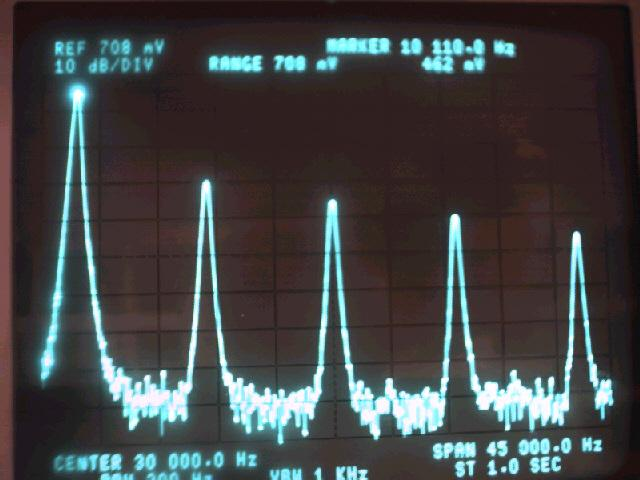20) Diagram 20: Frequency / Time View of Square Wave Modulation: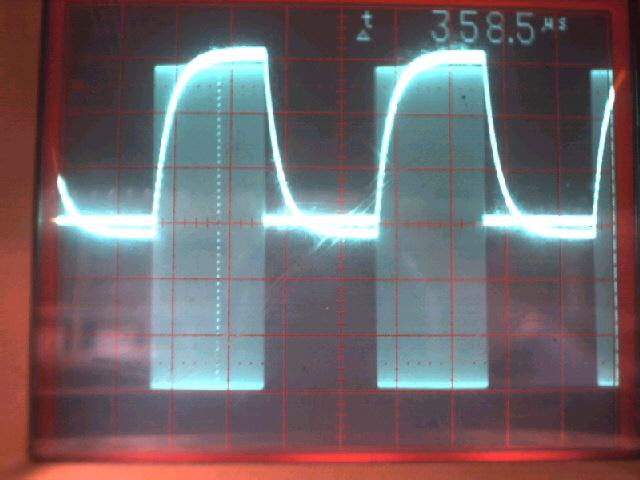21) Table 1: Harmonic Distortions of Various Modulation Indices:

Fundamental:

1st

2nd

3rd

4th

Total:

%Of Total:

10%:

58.8mV

40.2mV

19.3mV

12.7mV

14.9mV

87.1mV

75.2%

25%:

106mV

248mV

592mV

29.4mV

60.0mV

929.4mV

46.7%

50%:

221mV

684mV

1.07mV

38.8mV

102mV

1.8948mV

.850%

75%:

322mV

1.04mV

1.17mV

162mV

118mV

2.49mV

.767%

100%:

416mV

4.68mV

458mV

944mV

364mV

6.446mV

1.53%

120%:

462mV

4.06mV

141mV

2.78mV

58.8mV

7.0398mV

1.50%

22) Diagram 21: Voltage / Frequency View of Square Wave Modulation: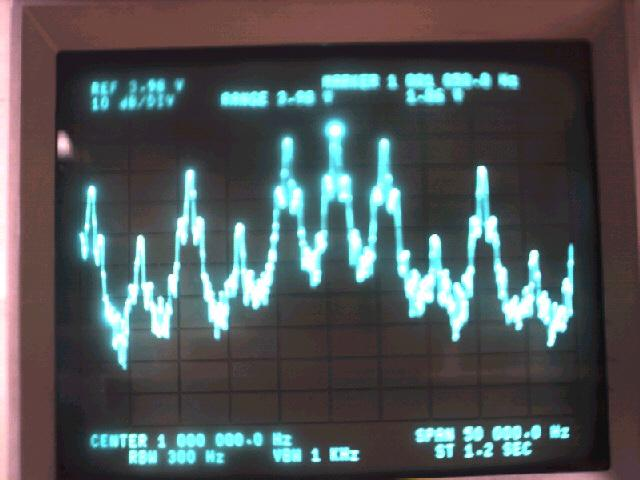23) Diagram 22: Voltage / Frequency View of Demodulated Output: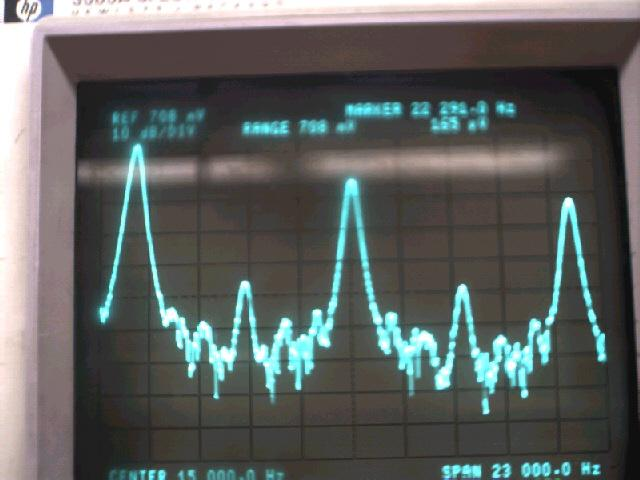24) Table 2: Harmonic Distortion of Demodulated Square Wave:

Fundamental:

1st

2nd

3rd

4th

Total:

% 0f Total:

Expected:

620mV

207mV

0

124mV

0

0

0

Observed:

620mV

406mV

141mV

278mV

58.8mV

0

0

Total:

0

199mV

141mV

154mV

58.8mV

353.2mV

0

% Of Total

0

32.9%

100%

35.6%

100%

0

36.3%

25) Diagram 23: Frequency / Voltage View of Triangle Wave Modulation: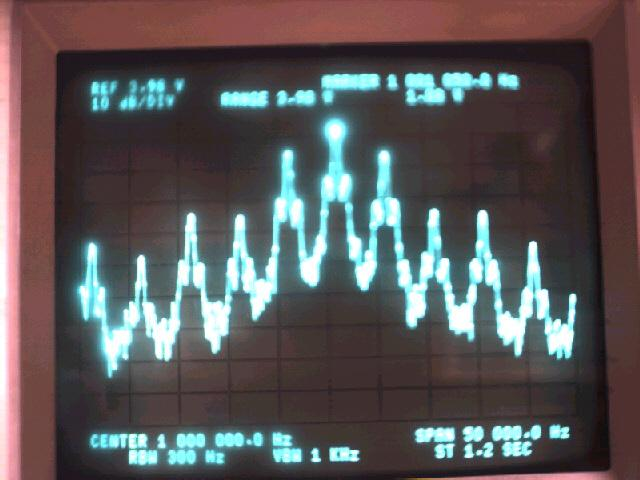26) Table 3: Harmonic Distortion of Triangle Wave:

Fundamental:

1st

2nd

3rd

4th

Total:

% 0f Total:

Expected:

406mV

0

45.1mV

0

16.2mV

0

0

Observed:

406mV

3.06mV

28.4mV

1.09mV

6.92mV

0

0

Total:

0

3.06mV

16.7mV

1.09mV

9.28mV

30.1mV

0

% Of Total:

0

100%

37.0%

100%

57.3%

0

6.91%

27) Diagram 24: Voltage / Time View of Triangle Wave Modulation: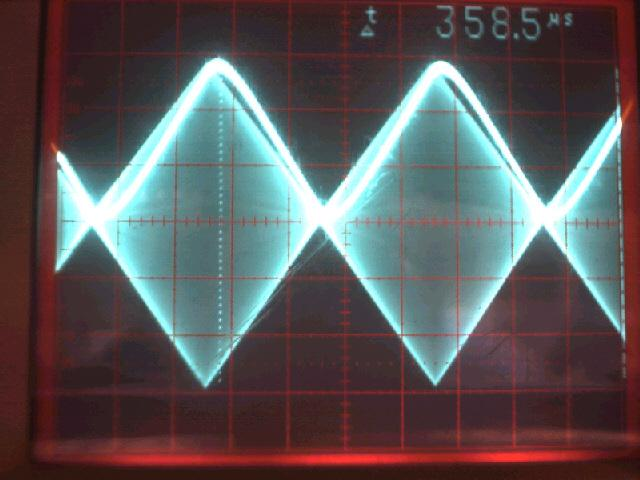28) Diagram 25: Voltage / Frequency View of Demodulated Output:Answers to Lab Questions

1) Q: Why was the power splitter used, and how does it operate?

A: It was used to divide the power between the two theoretical 50W outputs directly while still providing a 50W input to maintain maximum power transfer and keeping the SWR as close as possible to a theoretical 1. Otherwise, the Vx or Ix could become high from either a j or Z not being equal to 50W and damage the internal components. Note: Having an SWR not 1:1 also causes a situation called ground current which can cause RF burns. See attachments for more information.

2) Q: As the modulation percentage increased, what happened to the amount of harmonic distortion, why did this happen?

A: The distortion dropped off significantly because there was more of a reference signal and the diode was set into it's saturation point.

3) Q: Explain why the demodulated square wave and triangle wave had more distortion to them than the sine wave.

A: The waves had more distortion for multiple reason. The most significant being, that they require infinite bandwidth. Also, the signals could be processed differently because the signal nature and reactance of the modulator. Lastly there was no definitive way to ensure that the modulation index was perfectly 100%, it is possible that the modulation index was slightly higher than that and caused a significant amount of distortion in the signal, especially at overtones.

4) Q: What is the bandwidth required to send the following signal VIA DSB-SC: cos(2000pt) + cos(4000pt) + cos(6000pt)?

A: 12KHzConclusions

This lab has demonstrated some of the basic principles of DSB-FC modulation. Significant problems in the lab involved the fact that there wasn't a function generator capable of putting out a signal with enough voltage to obtain the required voltage. As a result, all specified voltages were divided by two during the actual experiment. Also, the generators were not designed for 50W loads, instead they were Hi-Z outputs (S100KW). As a result, the 50W resistor from the diode input to ground was removed during the experiment. Also, calculations are not compensated for harmonic distortions inherent to the function generators. That was somewhat discussed in the explanation of question 3. Other than that there were no other difficulties with this lab.Attachments

1. Original lab handout

2. Original lab data

3. Calculations